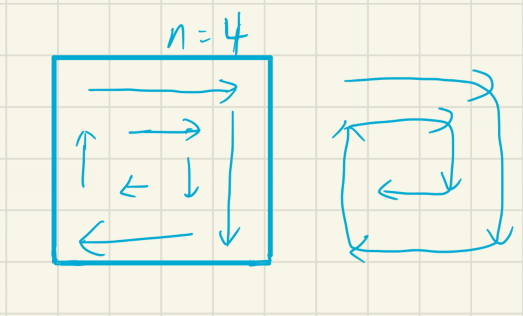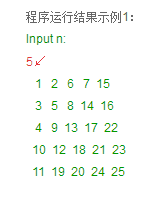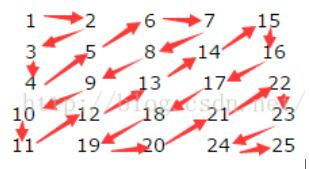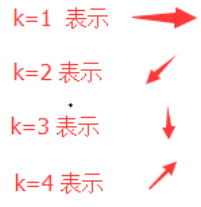• 给出一个不大于 9 的正整数 n，输出 n×n 的蛇形方阵。 从左上角填上 1 开始，顺时针方向依次填入数字，如同样例所示。注意每个数字有都会占用 3 个字符，前面使用空格补齐。 输入 4 输出 1 2 3 4 12 13 14 5 11 16 ...

题目描述
给出一个不大于 9 的正整数 n，输出 n×n 的蛇形方阵。

从左上角填上 1 开始，顺时针方向依次填入数字，如同样例所示。注意每个数字有都会占用 3 个字符，前面使用空格补齐。

输入
4
输出
1 2 3 4
12 13 14 5
11 16 15 6
10 9 8 7

思路:
方式1. 题目数据范围实在是太小了,可以直接采用打表的方式.
方式2. 矩阵依次是1 ~ n*n 的数, 从左往右,从上往下,再从右往左,下往上.
所以只要循环的方式可以实现"蛇形"就可以得到答案.Copy 方式 2 :

#include<stdio.h>
int a;    //这里定义全局变量,默认数组每个元素初始化为 0
int main(){
int n;
int k = 0; // 蛇形走位的初始值,每次循环成功 ++k;
scanf("%d",&n);
int x = 1,y = 0;

while( k < n*n){

// a[x][y+1] 是目标位置
//从左往右 , 判断如果没有超过边界并且目标位置没有被覆盖过则可以进行赋值
while( y < n  && a[x][y+1] == 0 )
{  a[x][++y] = ++k;}

//从上往下 ,
while( x < n  && a[x+1][y] == 0  )
{ a[++x][y] = ++k;}

//从右往左
while( y > 1 && a[x][y-1] == 0  )
{  a[x][--y] = ++k;}

//从下往上
while( x > 1  && a[x-1][y] == 0  )
{  a[--x][y] = ++k;}

}

for(int i = 1; i<= n; i++){
for(int j = 1; j <= n; j++){
printf("%3d",a[i][j]);
}
printf("\n");
}
return 0;
}

Copy 方式1:

#include <iostream>
using namespace std;
int n;
int main () {
cin >> n;
if(n == 1)
cout << "  1\n";
if(n == 2)
cout <<"  1  2\n  4  3\n";
if(n == 3) {
cout << "  1  2  3\n";
cout << "  8  9  4\n";
cout << "  7  6  5\n";
}
if(n == 4) {
cout << "  1  2  3  4\n";
cout << " 12 13 14  5\n";
cout << " 11 15 16  6\n";
cout << " 10  9  8  7\n";
}
if(n == 5) {
cout << "  1  2  3  4  5\n";
cout << " 16 17 18 19  6\n";
cout << " 15 24 25 20  7\n";
cout << " 14 23 22 21  8\n";
cout << " 13 12 11 10  9\n";
}
if(n == 6) {
cout << "  1  2  3  4  5  6\n";
cout << " 20 21 22 23 24  7\n";
cout << " 19 32 33 34 25  8\n" ;
cout << " 18 31 36 35 26  9\n";
cout << " 17 30 29 28 27 10\n";
cout << " 16 15 14 13 12 11\n";
}
if(n == 7) {
cout << "  1  2  3  4  5  6  7\n";
cout << " 24 25 26 27 28 29  8\n";
cout << " 23 40 41 42 43 30  9\n";
cout << " 22 39 48 49 44 31 10\n";
cout << " 21 38 47 46 45 32 11\n";
cout << " 20 37 36 35 34 33 12\n";
cout << " 19 18 17 16 15 14 13\n";
}
if(n == 8) {
cout << "  1  2  3  4  5  6  7  8\n";
cout << " 28 29 30 31 32 33 34  9\n";
cout << " 27 48 49 50 51 52 35 10\n";
cout << " 26 47 60 61 62 53 36 11\n";
cout << " 25 46 59 64 63 54 37 12\n";
cout << " 24 45 58 57 56 55 38 13\n";
cout << " 23 44 43 42 41 40 39 14\n";
cout << " 22 21 20 19 18 17 16 15\n";
}
if(n == 9) {
cout << "  1  2  3  4  5  6  7  8  9\n";
cout << " 32 33 34 35 36 37 38 39 10\n";
cout << " 31 56 57 58 59 60 61 40 11\n";
cout << " 30 55 72 73 74 75 62 41 12\n";
cout << " 29 54 71 80 81 76 63 42 13\n";
cout << " 28 53 70 79 78 77 64 43 14\n";
cout << " 27 52 69 68 67 66 65 44 15\n";
cout << " 26 51 50 49 48 47 46 45 16\n";
cout << " 25 24 23 22 21 20 19 18 17\n";
}
return 0;
}

展开全文c语言 c++ 算法
• 习6】蛇形方阵给出一个不大于 9 的正整数 $$n$$，输出 $$n\times n$$ 的蛇形方阵。从左上角填上 1 开始，顺时针方向依次填入数字，如同样例所示。注意每个数字有都会占用 3 个字符，前面使用空格补齐。无无输入 #14...

洛谷-P5731 【深基5.习6】蛇形方阵

给出一个不大于 9 的正整数 $$n$$，输出 $$n\times n$$ 的蛇形方阵。

从左上角填上 1 开始，顺时针方向依次填入数字，如同样例所示。注意每个数字有都会占用 3 个字符，前面使用空格补齐。

输入 #1

4

输出 #1

1 2 3 4

12 13 14 5

11 16 15 6

10 9 8 7

#include

using namespace std;

int a;

int main() {

int n, x=1, y=0, k=1;

scanf("%d", &n);

while (k <= n*n) {

while (y

while (x

while (y>1 && !a[x][y-1]) a[x][--y]=k++;

while (x>1 && !a[x-1][y]) a[--x][y]=k++;

}

for (int i=1; i<=n; ++i) {

for (int j=1; j<=n; ++j)

printf("%3d", a[i][j]);

printf("\n");

}

return 0;

}

展开全文洛谷p5731C语言
• #include<iostream> #include<cstdio> using namespace std; int main() { int n; cin>>n; int arr={0};//初始化数组 int x=1,y=0; int k=1; while(k<... wh.#include<iostream>
#include<cstdio>
using namespace std;

int main()
{
int n;
cin>>n;
int arr={0};//初始化数组
int x=1,y=0;
int k=1;
while(k<=n*n)
{
while(y<n && arr[x][y+1]==0)
{
arr[x][++y]=k++;
}
while(x<n && arr[x+1][y]==0)
{
arr[++x][y]=k++;
}
while(y>1 && arr[x][y-1]==0)
{
arr[x][--y] = k++;
}
while(x>1 && arr[x-1][y]==0)
{
arr[--x][y]=k++;
}
}
//格式化输出
for(int i=1;i<=n;i++)
{
for(int j=1;j<=n;j++)
printf("%3d",arr[i][j]);
printf("\n");
}
return 0;
}

展开全文• C语言实现蛇形矩阵

万次阅读 多人点赞 2017-12-19 21:37:14
c语言实现蛇形矩阵

题目如下图所示：解题思路分析：

我们按照图中标出的箭头指向，逐一的赋值整个矩阵。具体思路：

1. 初始的时候，行标i=0,列标j=0,data[i][j]=0,此时k = 1(k表示当前矩阵位置到下一位置的运动方向)；
2. 当data移动到下一个位置后，此时需要把k变为它当前位置的移动方向。
1) k = 1的时候，k的下一个运动方向可能是 2或者4;
A. data位于矩阵的第一行时，k变为2;
B. data位于矩阵的最下面一行时，k变为4.
2) k = 2的时候，k的下一个运动方向可能是 1或者2或者3;
A. data位于矩阵的第一列时，k变为3;
B. data位于矩阵的最下面一行时，k变为1；
C. data位于矩阵其它位置时，k仍为2.
3) k = 3的时候，k的下一个运动方向可能是 2或者4;
A. data位于矩阵的第一列时，k变为4;
B. data位于矩阵的最后一列时，k变为2.
4) k = 4的时候，k的下一个运动方向可能是1或者3或者4;
A. data位于矩阵的第一行时，k变为1;
B. data位于矩阵的最后一列时，k变为3；
C. data位于矩阵其它位置时，k仍为4.
此外，有左下顶角和右上顶角的位置需要额外关注。

主体代码如下：

int n;
int i = 0;  //行
int j = 0;  //列
int k = 1;  //运动方向. k =1 表示运动方向为从左往右移动;k=2 表示从右上角往左下角移动； k=3 表示向下移动 ；k=4 表示从左下角网右上角移动
int data ;
data = 1;
printf("Input n:\n");
scanf("%d",&n);
while( i!=n-1 || j !=n-1){
if(k == 1){
data[i][j+1] = data[i][j] + 1;
j = j + 1;
if(i == n-1){
k = 4;
}else{
k = 2;
}
}else if(k == 2){
data[i+1][j-1] = data[i][j] + 1;
j = j - 1;
i = i +1;
if( j == 0 && i != n-1){
k = 3;
}else if( i == n-1){
k = 1;
}else{
k = 2;
}
}else if(k == 3){
data[i+1][j] = data[i][j] + 1;
i = i+1;
if( j ==  n-1){
k = 2;
}else {
k = 4;
}
}else if(k == 4){
data[i-1][j+1] = data[i][j] + 1;
j = j + 1;
i = i - 1;

if(i == 0 && j != n-1){
k = 1;
}else if( j == n-1  )
{
k = 3;
}else{
k = 4;
}
}
}
//显示矩阵
for(int a=0;a<n;a++){
for(int b=0;b<n;b++){
printf("%d\t",data[a][b]);
}
printf("\n");
}

展开全文c语言 蛇形矩阵
• C语言输出蛇形矩阵

千次阅读 多人点赞 2020-06-09 01:35:20
写在前面:这里是小王成长日志，一名在校大学生，想在学习之余将自己...蛇形矩阵是由1开始的自然数依次排列成的一个矩阵上三角形。 输入 本题有多组数据，每组数据由一个正整数N组成。（N不大于100） 输出 对于每一组数.c语言 算法
• 编写程序，将1到n*n之间的每个整数，从1开始，按照顺序依次填入到n阶蛇形方阵（具体见样例）。 输入 在一行中给出一个正整数N（<100）。 输出 输出N×N的蛇形方阵。每行N个数字，每个数字占5位。 输入...c语言 开发语言 后端
• 蛇形矩阵的实现的经典案例for(i=1;i;i++)/*对副对角线上三角端点赋值*/ { k=k+i; if(i%2==1) { a[i]=k; a[i]=k+i; } else { a[i]=k; a[i]=k+i; } }蛇形矩阵
• /输入N，输出一个NN的蛇形矩阵 例如输入4，输出： 1 2 3 4 12 13 14 5 11 16 15 6 10 9 8 7 */ #include <stdio.h> int main(void) { //用户输入的值，创建n*n的矩阵 int n; //蛇形从1开始计数 ...
• 习6】蛇形方阵 题目描述 给出一个不大于 9 的正整数 nnn，输出 n×nn\times nn×n 的蛇形方阵。 从左上角填上 1 开始，顺时针方向依次填入数字，如同样例所示。注意每个数字有都会占用 3 个字符，前面使用空格补齐。...c语言 c++
• int main() { int i,j,a,n,k; printf("请输入列数n= "); scanf("%d",&n); k=1; //输出上三角 for(i=0; i<n;i++) { for(j=0; j<=i; j++) { if(...
• 【C语言】蛇形矩阵

千次阅读 2020-02-23 09:57:45
蛇形矩阵 题目描述 蛇形矩阵是由1开始的自然数依次排列成的一个矩阵上三角形。 输入 本题有多组数据，每组数据由一个正整数N组成。（N不大于100） 输出 对于每一组数据，输出一个N行的蛇形矩阵。两组输出之间不要...
• (即安什么便准来定义方阵的边长？)追问：只是要求打印如下方阵追答：#include#include void right(int i,int j,int n); //向右输入void down(int i,int j,int n); //向下输入void left(int i,int j,int n); //向左...c语言方阵编程
• 一、今天说的是哪种蛇形矩阵蛇形矩阵有多种，今天说的是下面这种回形的蛇形矩阵。如4x4的回形蛇形矩阵 如5x5的回形蛇形矩阵 通过分析发现，这种矩阵，在走一圈以后又变成一个小2个的矩阵，如5x5的矩阵，从1、2、3。...
• 习6】蛇形方阵 //#define LOCAL #include <iostream> #include <cstdio> #include <cstring> #include <cmath> #include <algorithm> #define inf 0x3f3f3f3f #define eps 1e-6 using...
• 需求如下： #include <stdio.h> #include <stdlib.h> #define N 100 int a[N][N] = {0}; int n = 0; typedef enum _MOVEDIR { move_rt = 1, //右上移动 ... bool bhwMove = fals
• 这是一个c语言的作业，能够输出逆时针蛇形矩阵，很好用！！C语言
• MAX），输出1到n蛇形矩阵。 【例如】 n=10 1 2 6 7 3 5 8 4 9 10 思路 昨天晚上无意看到这题，打算尝试一下。这种题无非就是找规律，和数学题差不多。当时没有工具，勉强在手机上的表格上写了蛇形数组，观察了下思路...蛇形数组 C语言 学习记录
• 编写程序，将1到n*n之间的每个整数，从1开始，按照顺序依次填入到n阶蛇形方阵（具体见样例）。 输入 在一行中给出一个正整数N（<100）。 输出 输出N×N的蛇形方阵。每行N个数字，每个数字占5位。 输入...c语言 开发语言 后端
• 从键盘任意输入一个自然数n（n表示矩阵的大小，假设不超过100），请编程输出一个nn的蛇形矩阵。如果输入的n不是自然数或者输入了不合法的数字，则输出"Input error!"。 提示 用两个双重循环分别计算nn矩阵...
• 方阵填数：在一个nn的数组中，填入1，2，3，….NN个数，并要求按如下格式输出该数组的元素，例如一个5*5的数组输出顺序为： 13 14 15 16 1 12 23 24 17 2 11 22 25 18 3 10 21 20 19 4 9 8 7 6 5 分析 ...
•蛇形矩阵
• 重点在于蛇形方阵的填充过程，期间填充方向循环着：右/下/左/上···。 注意*每个数字占用三个字符的表达方式—— %3d 。 代码： #include<stdio.h> void fill(int snake[],int n); int main() { int n;...
• #include&lt;stdio.h&gt; int main() { int a = { 1 }, n, i = 0, j = 0, k = 1;... //k=1表示向左走，k=2表示从右上角向左下角走 //k=3表示向下走，k=4表示从左下角向右上角走 ...
• C语言每日小练(二)——蛇形数组例：在n*n方阵里输入1,2,3,...,n*n，要求填成蛇形。例如n=4时方阵为：10 11 12 19 16 13 28 15 14 37 6 5 4上面的方阵中，多余的空格知识为了便于观察矩阵，不必严格输出，n<=8。解......

蛇形方阵c语言c语言 订阅